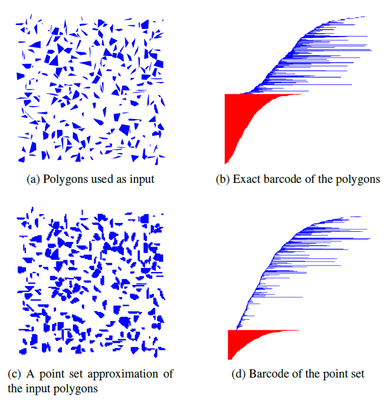# The Offset Filtration of Convex Objects

## Abstract

We consider offsets of a union of convex objects. We aim for a filtration, a sequence of nested cell complexes, that captures the topological evolution of the offsets for increasing radii. We describe methods to compute a filtration based on the Voronoi partition with respect to the given convex objects. We prove that, in two and three dimensions, the size of the filtration is proportional to the size of the Voronoi diagram. Our algorithm runs in $\Theta(n \log{n})$ in the 2-dimensional case and in expected time $O(n^{3 + \epsilon})$, for any $\epsilon > 0$, in the 3-dimensional case. Our approach is inspired by alpha-complexes for point sets, but requires more involved machinery and analysis primarily since Voronoi regions of general convex objects do not form a good cover. We show by experiments that our approach results in a similarly fast and topologically more stable method for computing a filtration compared to approximating the input by point samples.Figure: A comparison between an exact barcode and an approximated barcode. An illustration of polygons used as input for our experiments is shown in (a). There are~250 polygons with a total of~1060 vertices, inside a square of side length~100. The exact barcode of the polygons, computed using the restricted nerve method, is shown in (b). Red bars represent connected components and blue bars represent holes. It can be observed that all connected components are born at offset 0, since no connected components are created as the offset increases. When increasing the offset, connected components merge and therefore die, and holes are created and then die as they get filled. (c) shows an illustration of a point set approximation of the input polygons, with $\epsilon = 0.5$. Each point is displayed as a pixel of size $\sqrt{2}\epsilon$. In (d) we see the barcode of the approximation point set, which is an approximation of the exact barcode of the polygons. The approximated barcode contains many more bars compared to the exact barcode. Noise, in the form of short bars, can be observed throughout the entire barcode.

• Dan Halperin, Michael Kerber and Doron Shaharabani
The Offset Filtration of Convex Objects
European Symposium on Algorithms (ESA 2015), to appear;
Arxiv